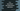# C++ Increment and decrement operators## C++ Increment and decrement operators:

Increment and decrement operators are most commonly used operators in most programming languages like C, C++, Java etc. These are defined by ++ for increment and for decrement.

The increment operator adds 1 and the decrement operator subtracts 1.

There are two more ways to use these operators: prefix and postfix.

In this post, we will learn how to use these operators, and how prefix and postfix versions works.

### Prefix and Postfix versions:

Both increment and decrement operators can be used as:

• Pre-Increment (++e)
• Pre-Decrement (—e)
• Post-Increment (e++)
• Post-Decrement (e—)

For pre-increment or pre-decrement, the value is first incremented or decremented by 1 and it returns a reference to the result.

For post-increment or post-decrement, it returns the value first and then it increments or decrements the value by 1.

## Example of pre and post increment/decrement:

Let’s create an example for pre and post increment.

``````#include <iostream>

using namespace std;

int main()
{
int firstVar = 1;
int secondVar = 1;

cout << "++firstVar: " << ++firstVar << endl;
cout << "secondVar++: " << secondVar++ << endl;

cout << "firstVar: " << firstVar << endl;
cout << "secondVar: " << secondVar << endl;

return 0;
}``````

Here,

• firstVar and secondVar are integer variables and these are initialized as 1.
• The first two statements are printing the values of firstVar and secondVar while doing pre and post increment.
• The last two statements are printing the values after the increment is done for the variables.

If you run this program, it will print the below output:

``````++firstVar: 2
secondVar++: 1
firstVar: 2
secondVar: 2``````

As you can see, for pre-increment, it returns the incremented value and for post-increment, it returns the value at current state.

Once the increment is done, it prints the same values for both.

### Example of pre and post decrement:

We can change the above program to use with pre and post decrement as well. For example:

``````#include <iostream>

using namespace std;

int main()
{
int firstVar = 1;
int secondVar = 1;

cout << "--firstVar: " << --firstVar << endl;
cout << "secondVar--: " << secondVar-- << endl;

cout << "firstVar: " << firstVar << endl;
cout << "secondVar: " << secondVar << endl;

return 0;
}``````

Similar to the above example, it will print:

``````--firstVar: 0
secondVar--: 1
firstVar: 0
secondVar: 0``````

As you can see, for pre-decrement the return value is the final value, i.e. 0 and for post-decrement, the return value is the value at current state.